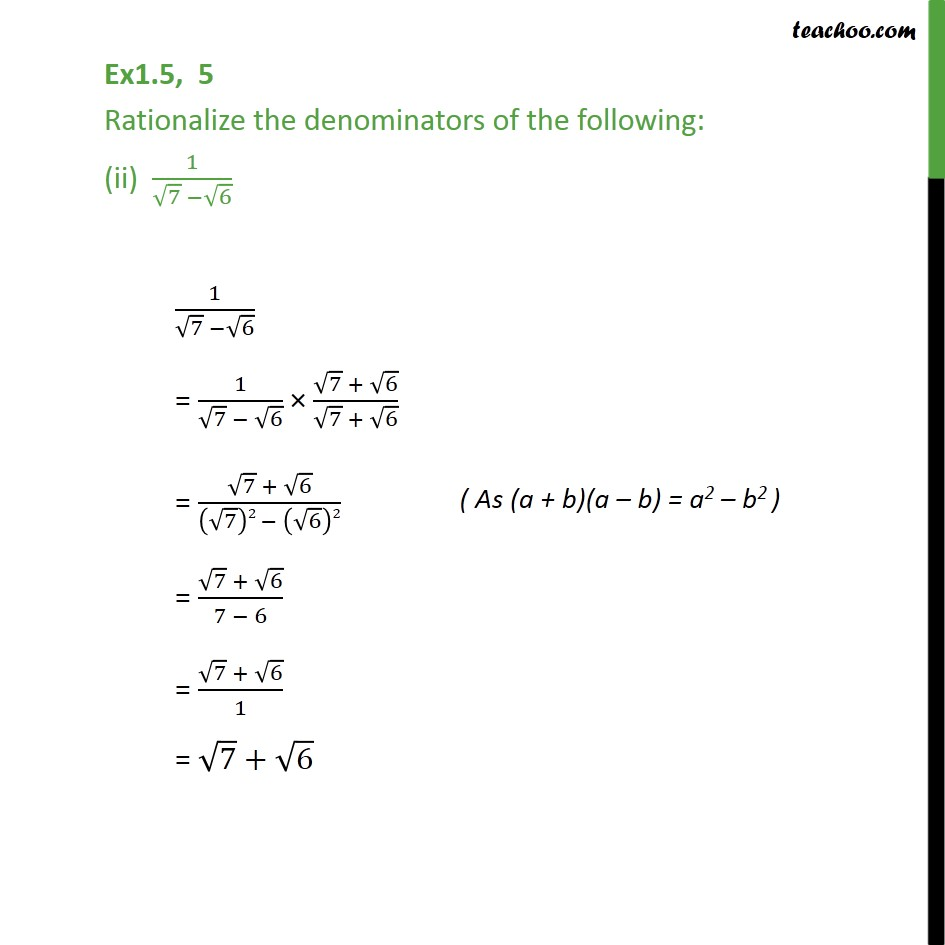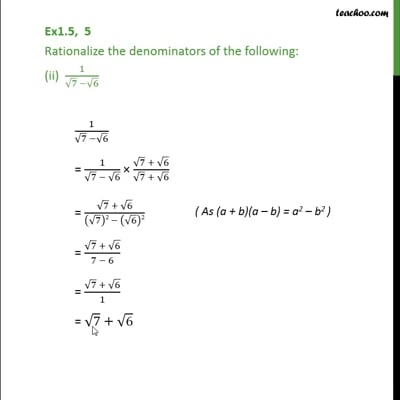Ex 1.5

Chapter 1 Class 9 Number Systems
Serial order wiseThis video is only available for Teachoo black users

Maths Crash Course - Live lectures + all videos + Real time Doubt solving!

### Transcript

Ex1.5, 5 Rationalize the denominators of the following: (ii) 1/(√7 −√6) 1/(√7 −√6) = 1/(√7 − √6) × (√7 + √6)/(√7 + √6) = (√7 + √6)/((√7)2 − (√6)2) = (√7 + √6)/(7 − 6) = (√7 + √6)/1 = √7+√6